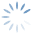Help OnLine | Options Strategy – Montecarlo Simulations
3561

# Options Strategy – Montecarlo Simulations

### Understand the Montecarlo Simulations function

Click on the image to expand

This feature allows you to draw estimates through simulations using the Montecarlo Method . The window consists mainly of two sections, the Simulation Parameters section and the section with the related graphics.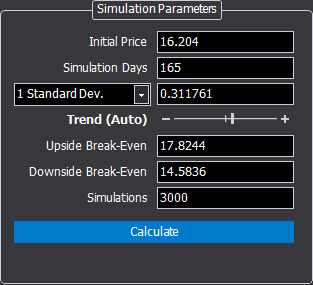• Initial Price: starting price from which launches will be made;
• Simulation Days: duration in days of the simulation;
• 1 Standard Deviations / Historical Volatility: value of 1 standard deviation relative to the price trend of the underlying calculated over 21 days or historical volatility;
• Trend (Auto): the trend of the underlying is automatically detected to have a likely simulation, however this parameter can be modified at will by manually positioning the cursor in the central position (simulation in the absence of trend and therefore symmetrical), or with more or less trend based on their own assessment;
• Upside Break-Even: upper limit with which to check the probability percentages;
• Downside Break-Even: lower limit with which to check the probability percentages;
• Simulations: number of launches that will be performed. Remember that 25,000 launches are the minimum measure to obtain statistically reliable results.

If the Montecarlo Simulation is started from the strategy, the fields are automatically filled in with the values of the strategy, where available.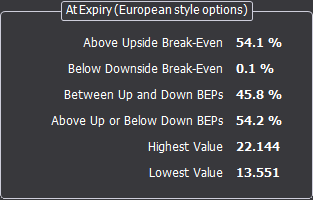• Above Upside Break-Even: indicates the percentage of probability that at expiry, the price of the underlying is higher than the Upside Break-Even Point;
• Below Downside Break-Even: indicates the percentage of probability that the price of the underlying will be lower than the Downside Break-Even Point at expiry;
• Between Up and Down BEPs: indicates the percentage of probability that at expiry, the price of the underlying is included in the Break-Even Points;
• Above Up or Below Down BEPs: indicates the percentage of probability that the price of the underlying will be out of Break-Even Points at expiry;
• Highest Value: indicates the highest value reached by the simulation;
• Lowest Value: indicates the lowest value reached by the simulation.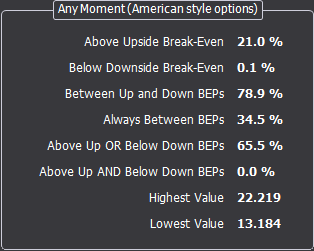• Above Upside Break-Even: indicates the percentage probability that the price of the underlying is higher than the Upside Break-Even Point in the time frame set in Simulation Days;
• Below Downside Break-Even: indicates the probability percentage that the price of the underlying is lower than the Downside Break-Even Point in the time frame set in Simulation Days;
• Between Up and Down BEPs: indicates the probability percentage that the price of the underlying is between the Break-Even Points in the time frame set in Simulation Days;
• Always Between BEPs: indicates the probability percentage that the price of the underlying is always included between the Break-Even Points in the time frame set in Simulation Days;
• Above Up OR Below Down BEPs: indicates the probability percentage that the price of the underlying was at least once higher than the Upside Break-Even Point OR  less than the Downside Break-Even Point in the time frame set in Simulation Days;
• Above Up AND Below Down BEPs: indicates the probability percentage that the price of the underlying was at least once higher than the Upside Break-Even Point AND  less than the Downside Break-Even Point in the time frame set in Simulation Days;
• Highest Value: indicates the highest value reached by the simulation;
• Lowest Value: indicates the lowest value reached by the simulation.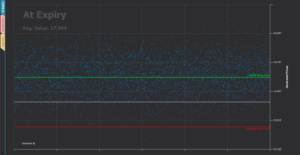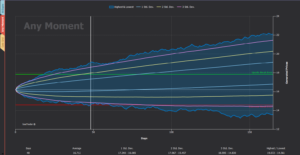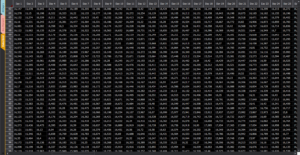This chart shows the launches of the Montecarlo Simulation performed, where each launch corresponds to a dot. The expiration price of the simulation is then displayed, which together with the Break-Even provides an immediate visual perception of the simulation carried out and of what is the price at expiry.

This graph generates the percentages shown in the At Expiry box.

In this graph, on the other hand, the maximum and minimum are indicated for each day of the simulation, and not only at the end of the simulation, in this way we have the perception of the deviation during the life of the simulation.

This graph generates the percentages shown in the Any Moment box.

The table shows the prices generated by the launches set for each day of the simulation.